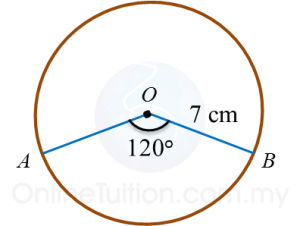# 5.1b Circles

5.1.2 Circumference of a Circle

Example:
Calculate the circumference of a circle with a diameter of 14 cm. $\left(\pi =\frac{22}{7}\right)$

Solution:

5.1.3 Arc of a Circle
The length of an arc of a circle is proportional to the angle at the centre.

Example:Calculate the length of the minor arc AB of the circle above.
$\left(\pi =\frac{22}{7}\right)$

Solution:

5.1.4 Area of a Circle

Example:
Calculate the area of each of the following circles that has
(a) a radius of 7 cm,
(b) a diameter of 10 cm.
$\left(\pi =\frac{22}{7}\right)$

Solution:
(a)

(b)

5.1.5 Area of a Sector
The area of a sector of a circle is proportional to the angle at the centre.

Example: